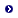# Beifang Chen

### Department of Mathematics Hong Kong University of Science and Technology Clear Water Bay, Kowloon Hong Kong

 Tel: (852) 2358-7449 Fax: (852) 2358-1643 Email:

Dr. Chen is Professor of Mathematics in HKUST and guest Professor in Nankai University. He was an applied mathematics instructor in Massachusetts Institute of Technology before he joined HKUST in Fall 1993.Research InterestsCombinatorics: Enumerative combinatorics, partially ordered sets, group arrangements, polytopes and polyhedra.Graph Theory: Graph polynomials, Graph learning, graph convolutional network, graph database, knowledge graphs.Topology/Geometry: Subspace arrangements, cellular spaces, semi-algebraic sets, toric variety,  weight homology.

CoursesMath3343

-----Math113

-----Math111

-----Math2343

-----Math2721

-----Math4221

-----Math4821B

-----Math5380

-----

PoemsSwing a Little-Boat-1.pdflittle boatPreprintsPublications

2006--2010:

The flow and tension spaces and lattices of signed graphs.A bijection for Eulerian-equivalence classes of totally cyclic orientations. (with Arthur L.B. Yang and Terence Y.J. Zhang) , Graphs Combin. 24 (2008), 519--530.Gauss-Bonnet formula, finiteness condition, and asymptotic characterization for graphs embedded in surfaces. (with Guantao Chen) , Graphs Combin. 24 (2008), 159--183.

2001--2005:Problems from the Cottonwood Room (with Beck, M; Fukshansky, L.; Haase, C.; Knutson, A.; Reznick, B.; Robins, S. and Schürmann, A.), in: Integer Points in Polyhedra -- Geometry, Number Theory, Algebra, Optimization, 179-191, Contemp. Math., 374, Amer. Math. Soc., Providence, RI, 2005.Ehrhart polynomials of lattice polyhedral functions, in: Integer Points in Polyhedra -- Geometry, Number Theory, Algebra, Optimization, 37-63, Contemp. Math., 374, Amer. Math. Soc., Providence, RI, 2005.A simplified elementary proof of Hadwiger's volume theorem, Geometriae Dedicata, 105(2004), 107-120.The Pick theorem and the proof of the reciprocity law for Dedekind sums, Annals Combin., 7(2003), 425-439.On semi-Eulerian partially ordered sets with boundary (with Lau, M.), European J. Combin., 24(2003), 955-968.Lattice points, Dedekind sums, and Ehrhart polynomials of lattice polyhedra, Discrete & Computational Geometry, 28(2002), 175-199.Generalizations of Knopp's identity (with Sun, Z.W.), J. Number Theory, 97(2002), 186-198.Graph homotopy and Graham homotopy (with Yau, S.-T. and Yeh, Y.-N.), Discrete Mathematics, 241(2001), 153-170.

1996---2000:Counting lattice points of rational polyhedra (with Turaev, V.), Advances in Mathematics, 155(2000), 84-97.On characteristic polynomials of subspace arrangements, Journal of Combinatorial Theory Series A, 90(2000), 347-352.Minkowski Algebra I: A convolution theory of closed convex sets and relatively open convex sets, Asian Journal of Mathematics, 3(1999), 609-634.A note on cyclic colorations and minimal triangulations (with Lawrencenko, S.), Yokohama math. J., 47(1999), 93-99.Eulerian 2-strata spaces (with Yan, M.), Journal of Combinatorial Theory Series A, 85(1999), 1-28.Domination Number and Neighborhood Conditions (with Zhou, S.M.), Discrete Mathematics, 195(1999), 81-91.Eulerian Stratification of Polyhedra (with Yan, M.), Advances in Applied Mathematics, 21(1998), 22-57.Singularity from Eulerian viewpoint (with Yan, M.), Proceedings of Steklov Mathematical Institute, 221(1998), 296-311.Structural Characterization of Projective Flexibility (with Lawrencenko, S.), Discrete Mathematics, 188(1998), 233-238.Weight Functions, Double Reciprocity Laws, and Volume Formulas for Lattice Polyhedra, Proceedings of the National Academy of Sciences of the United States of America, 95(1998), 9093-9098.The Geometric Cone Relations for Simplicial and Cubical Complexes (with Yan, M.), Discrete Mathematics, 183(1998), 39-46.The Upper Bounds for f-Domination Numbers of Graphs (with Zhou, S.M.), Discrete Mathematics, 185(1998), 239-243.Linear Conditions on the Number of Faces of Manifolds with Boundary (with Yan, M.), Advances in Applied Mathematics, 19(1997), 144-168.Pansystems Optimization, Generalized Principles of Optimality, and Fundamental Equations of Dynamic Programming, Kybernetes, 26(1997), 316-333.Parametric Möbius Inversion Formulas, Discrete Mathematics, 169(1997), 211-215.Geometric Dehn-Sommerville Relations, Journal of Combinatorial Theory, Series A, 73(1996), 142-151.The Incidence Algebra of Polyhedra over the Minkowski Algebra, Advances in Mathematics, 118(1996), 337-365.

1991---1995:The Mixed Volumes and Geissinger Multiplications of Convex Sets, Studies in Applied Mathematics, 91(1994), 39-50.Totally Invariant Set Functions of Polynomial Type (with Rota, G.-C.;), Communications on Pure and Applied Mathematics, 47(1994), 187-197.Some Explanations of Dobinski's Formula (with Yeh, Y.N.), Studies in Applied Mathematics, 92(1994), 191-199.The Induced Linear Maps on Minkowski Algebras of Convex Sets, Proceedings of the First International Tainan-Moscow Workshop (1994), (Fong, Y., Knauer, U. & Mikhalev, A.V., eds.), pp. 181-198., Water de Gruyter & Co., Berlin, 1996.On the Euler Characteristics of Finite Unions of Convex Sets, Discrete and Computational Geometry, 10(1993), 79-93.The Gram-Sommerville and Gauss-Bonnet Theorems and Combinatorial Geometric Measures for Noncompact Polyhedra, Advances in Mathematics, 91(1992), 269-291.

1983---1990:Some Pansystem Models of General Equilibrium in Economics (with Wu, X.M.), Kybernetes, 14(1985), 37-43.Pansystems Clustering Method and Its Application to Classification of Fuzzy things, Fuzzy Mathematics, 3(1984), 51-58.Pansystems Investigation of ε-equilibrium under Fuzzy Preferences, Fuzzy Mathematics, 4(1984), 93-98.Quasi-transitivity and Problem of Social Choice (with Wu, S.Z.), Science Exploration, 4(1984), 47-52.On the Problem of Equilibrium under Quasi-transitive Preferences (with Wu, S. Z.), Exploration of Nature, 1(1984), 29-36.Pansystems Investigations of Quotient Systems and Its Application, Science Exploration, 4(1983), 81-88.Some Logic Transforming Conservation of Semi-equivalence Pansystems Theory, Science Exploration, 3(1983), 87-94.